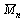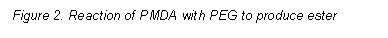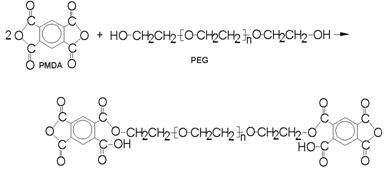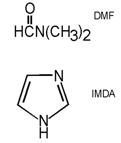HOME | INTRODUCTION | PRELAB | PROCEDURE | REPORT | PRINTABLE PDFPolymer End Group Analysis - The Determination of Average Molecular Weight: Introduction

Polymers

A polymer, a type of macromolecule, is a compound of high molecular weight formed by combining a large number of small molecules. The small molecules, called monomers, may all be of one type, as in the compound used in this experiment, or may be of different types.
Polymers are very important in biological systems. For example, proteins are composed of intricate sequences of amino acids, and polysaccharides contain repeating units of simple sugar molecules. Our everyday lives are also greatly influenced by polymers that are not obtained from natural sources. Synthetic polymers are known to us as various fibers (e.g., Dacron), plastics (e.g., polyvinyl chloride abbreviated PVC and polystyrene), and elastomers. See Fig. 1.
Like PVC and polystyrene, the compound involved in this experiment is synthesized from only one kind of monomer molecule, ethylene glycol. The monomers are joined by elimination of a molecule of water to form a series of ether linkages.The polymer is called either poly(ethylene glycol) (PEG for short), poly(ethylene oxide), or, more properly, poly(oxyethylene). PEG is commonly found in the list of ingredients in hair preparations and cosmetics. It is a good lubricant and also has the desirable property of being soluble in water. Therefore, PEG is heavily used as a base for therapeutic ointments, and it is used industrially as a lubricant in the formation of textile fibers and metal products.Molecular Weight The physical properties of polymers depend heavily on their molecular weights, which vary according to the number of repeating monomer units (the n’s in the structures in Fig. 1) per polymer molecule. For example, the PEG molecules to be studied in this experiment have molecular weights less than 1000 and are viscous liquids. As the molecular weight increases, the compound is more grease-like, and, if the MW is greater than about 6000, PEG is a white solid.
The use of the term “molecular weight” is somewhat misleading, because it implies that a sample of a polymer has a uniform formula weight (i.e., that n is the same for all the polymer molecules in a sample). For many biopolymers, most notably proteins, the sequence of the monomers is dictated by the specific biological role of the compound, and the structure and molecular weight is the same for all molecules of that type. However, whenever a synthetic polymer is made, chains of varying length (varying n’s) are produced, and the product has a range of formula weights. Therefore, the “molecular weight” is really an average for the sample. To complicate the situation, there are several types of averages, depending on how the molecular weight is measured.
In this experiment the number average molecular weight,, of a sample of poly(ethylene glycol) will be determined. Conceptuallyis the simplest of the molecular weights, because it corresponds to the usual notion of an average. The weights of all the molecules are added together and then the sum is divided by the total number of molecules present. In symbolic formis given bywhere Ni is the number of moles of polymer with molecular weight Mi.
In the numerator each contributing molecular weight in the sample, called Mi, is multiplied by the corresponding number of moles, Ni. The sum is then divided by the total number of moles in the sample. Unfortunately, it is usually not possible to count up the number of moles of each chain length, and the value of Mn must be obtained indirectly. You should recognize that the numerator is actually the total mass of the sample in grams, and, as stated above, this is divided by the total number of moles in the sample. Therefore, the number average molecular weight may be determined experimentally by measuring the total number of moles of polymer in a known weight of sample.
Determining Number of Moles To determine the number of moles of polymer the analysis method must respond equally to each molecule without regard to its chain length. As the structures given above show, the monomer molecules on the ends of the chain must in some way be different from the chain itself. For a linear polymer (i.e., one in which there are no bonds, called crosslinks, between the chains) every molecule has two end groups, which may be identical, as in PEG, or different, as in Dacron. (For polymers such as PVC and polystyrene the identities of R and R’ depend on the choice of reagent used to initiate the polymerization reaction.) Thus, if the end groups can be analyzed, the number of polymer molecules may be calculated using simple stoichiometric relationships. This method for determiningis called end group analysis.Some Specifics The ends of PEG are alcohol groups, which may be analyzed by a reaction known as esterification. In an esterification the -OH reacts with an organic acid, or, more commonly, with a more reactive derivative of the acid. In this experiment the anhydride of 1,2,4,5-benzenetetracarboxylic acid, known commonly as pyromellitic dianhydride, or PMDA for short, will be used. The reaction with PEG is shown in Fig. 2.
The reaction must be conducted in a nonaqueous solvent, and, because of its low volatility and desirable solvating properties, N,N-dimethyl formamide, DMF, is a good choice. A catalyst is also required, and in many esterification procedures pyridine, a rather foul-smelling organic base, is used. However, for the reaction of alcohols with PMDA it has been found that imidazole, IMDA, a very soluble solid with no odor, has at least equal catalyzing power. The formulas of DMF and IMDA are shown below.
The analysis involves an indirect titration procedure. An excess of PMDA is mixed with the weighed PEG sample, and the catalyst is added. After the reaction is complete (at least one-half hour reaction time), water is added to convert the unreacted anhydride groups to the acid form, and the acid is titrated with standard NaOH.The equations in Fig. 3 show that each ester linkage to a PEG end group replaces one of the four acids formed from the PMDA, and, as a result, the number of moles of titratable protons decreases as the number of end groups increases. If the total number of moles of PMDA added at the start is known, the number of moles of -OH end groups may be calculated from the volume of base needed for the titration.
A standard solution of PMDA in DMF cannot be prepared directly by weighing and dilution. The PMDA solution must be standardized by reacting a known volume with water and titrating with base. It should be noted that the imidazole catalyst is itself a base, but it is so weak that it does not cause an error in the titration. For consistent results, however, an equal amount of the catalyst should be added to all samples.Figure 3. Formation of acid by reaction with water

Another Molecular Weight As discussed above, there is more than one expression for the average molecular weight of a polymer. The weight average molecular weight,, is given bywhere Wi is the weight of polymer with molecular weight Mi. Here each M is multiplied by the weight present in the sample, and the sum of all these contributions is divided by the total weight of the sample. Unless the sample consists of only one chain length,is always greater than. The ratio oftois called the polydispersity, and is an indication of the breadth of the distribution of molecular weights in the sample.